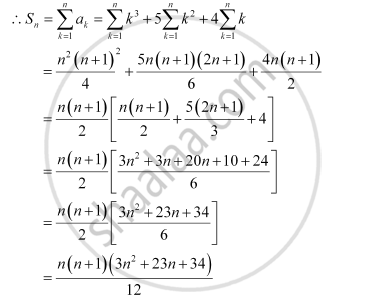CBSE (Arts) Class 11CBSE
Share
Notifications

View all notifications
Books Shortlist
Your shortlist is empty

# Find the Sum to N Terms of the Series Whose Nth Term is Given by N (N + 1) (N + 4). - CBSE (Arts) Class 11 - Mathematics

Login
Create free account

Forgot password?
ConceptSum to N Terms of Special Series

#### Question

Find the sum to n terms of the series whose nth term is given by n (n + 1) (n + 4).

#### Solution

an = n (n + 1) (n + 4) = n(n+ 5n + 4) = n3 + 5n2 + 4nIs there an error in this question or solution?

#### APPEARS IN

NCERT Solution for Mathematics Textbook for Class 11 (2018 to Current)
Chapter 9: Sequences and Series
Q: 8 | Page no. 196
Solution Find the Sum to N Terms of the Series Whose Nth Term is Given by N (N + 1) (N + 4). Concept: Sum to N Terms of Special Series.
S